# Enzyme Inhibition

Michaelis-Menton (MM) KineticsCompetitive Inhibiton
 [E] + [S]
 k1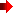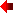k2
 [ES]
 k3[E] + [P]
Assumptions and givens:
• d[ES]/dt = 0 -- steady-state approximation
• [P] = 0 -- at time zero
• V = d[P]/dt = k3 [ES]
• [Etotal] = [E] + [ES]
• Vmax = k3 [Etotal]
• KM = (k2+ k3)/k1= [S]1/2
where V = (1/2) Vmax
V / Vmax = [S] / ([S] + KM ) -- MM equation
 [E] + [S] + [I] kI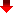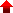k-I [EI]
 k1k2
 [ES]
 k3[E] + [P]
Assumptions and givens:
• d[ES]/dt = 0 -- steady-state approximation
• [P] = 0 -- at time zero
• V = d[P]/dt = k3 [ESn]
• [Etotal] = [E] + [ES] + [EI]
• Vmax = k3 [Etotal]
• KM = (k2+ k3)/k1
• KMapp = KM ( 1 + [I] / KI ) = ([S]1/2) where V = (1/2) Vmax
V / Vmax = [S] / ([S] + KMapp )

Noncompetitive InhitionUncompetitive Inhibiton
 [E] + [S] + [I] kIk-I [EI]
 k1k2
 [ES] + [I] kIk-I [ESI]
 k3[E] + [P]
Assumptions and givens:
• d[ES]/dt = 0 -- steady-state approximation
• [P] = 0 -- at time zero
• V = d[P]/dt = k3 [ESn]
• [Etotal] = [E] + [ES] + [EI] + [ESI]
• Vmax = k3 [Etotal]
• Vmaxapp = Vmax / ( 1 + [I] / KI )
• KM = (k2+ k3)/k1= ([S]1/2)
where V = (1/2) Vmaxapp
V / Vmaxapp = [S] / ([S] + KM )
 [E] + [S]
 k1k2
 [ES] + [I] kIk-I [ESI]
 k3[E] + [P]
Assumptions and givens:
• d[ES]/dt = 0 -- steady-state approximation
• [P] = 0 -- at time zero
• V = d[P]/dt = k3 [ESn]
• [Etotal] = [E] + [ES] + [ESI]
• Vmax = k3 [Etotal]
• Vmaxapp = Vmax / ( 1 + [I] / KI )
• KM = (k2+ k3)/k1
• KMapp = KM / ( 1 + [I] / KI ) = ([S]1/2) where V = (1/2) Vmaxapp
V / Vmaxapp = [S] / ([S] + KMapp )

Continue the kinetic analysis of enzymes by opening the following Excel spreadsheet

Graphical Analysis of Enzyme Inhibition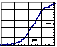Return to Biochemistry 108A Home PagePress the browser's "Back" key to return to previous page.Search for a Specific Topic© Duane W. Sears Revised: July 27, 1998